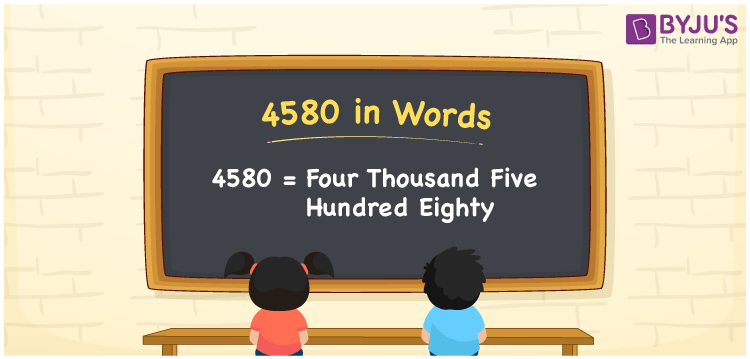# 4580 in words

4580 in words is written as Four Thousand Five Hundred and Eighty. 4580 represents the count or value. The article on Counting Numbers can give you an idea about count or counting. The number 4580 is used in expressions that relate to money, distance, length and others. Let us consider an example for 4580. ”Her employee number is Four Thousand Five Hundred and Eighty”.

 4580 in words Four Thousand Five Hundred and Eighty Four Thousand Five Hundred and Eighty in Numbers 4580

## 4580 in English Words## How to Write 4580 in Words?

We can convert 4580 to words using a place value chart. The number 4580 has 4 digits, so let’s make a chart that shows the place value up to 4 digits.

 Thousands Hundreds Tens Ones 4 5 8 0

Thus, we can write the expanded form as:

4 × Thousand + 5 × Hundred + 8 × Ten + 0 × One

= 4 × 1000 + 5 × 100 + 8 × 10 + 0 × 1

= 4580

= Four Thousand Five Hundred and Eighty.

4580 is the natural number that is succeeded by 4579 and preceded by 4581.

4580 in words – Four Thousand Five Hundred and Eighty.

Is 4580 an odd number? – No.

Is 4580 an even number? – Yes.

Is 4580 a perfect square number? – No.

Is 4580 a perfect cube number? – No.

Is 4580 a prime number? – No.

Is 4580 a composite number? – Yes.

## Solved Example

1. Write the number 4580 in expanded form

Solution: 4 × 1000 + 5 × 100 + 8 × 10 + 0 × 1

We can write 4580 = 4000 + 500 + 80 + 0

= 4 × 1000 + 5 × 100 + 8 × 10 + 0 × 1

## Frequently Asked Questions on 4580 in words

Q1

### How to write 4580 in words?

4580 in words is written as Four Thousand Five Hundred and Eighty.
Q2

### Is 4580 a perfect square number?

No. 4580 is not a perfect square number.
Q3

### Is 4580 a prime number?

No. 4580 is not a prime number.